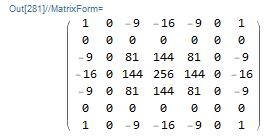# Cubic interpolation (or: how to get the filter matrix?)

So I’ve been trying to wrap my head around this particular piece of information:

I understand what h_l^T h_l and h_c^T h_c do and how to apply the resulting matrices. However, I do not understand how the author arrived at the ‘unseparable’ matrices in (3.6). Reading the references paper  did not help too much.

Is anyone here who can clear up the confusion, and possibly show me how this could be extended to even larger filters (just for fun)?

@Thanatomanic Roel, is the 7x7 kernel in 3.6) meant to be for the green channel of a bayer array?
If that’s the case, It looks wrong… and the 3x3 kernel as well

According to the source, yes, it is supposed to be for a typical Bayer CFA. The 3x3 kernel seems allright (cf. here section 1.2.2).

Edit: The kernel in (3.6) is for the green channel. The one for the red/blue channel can be computed by doing h_c^T h_c using h_c from (3.5).But the green channel has this layout:

`gr` means green, `ng` means non green

``````ng gr ng gr ng
gr ng gr ng gr
ng gr ng gr ng
``````

Edit: Or was that for compressed channels, but then I don’t understand why there are zero weightings around the 3x3 centre block…

Edit: the red/blue channels have this layout (if not compressed):
Example for blue: `bl` means blue, `nb` means non blue:

``````bl nb bl nb bl
nb nb nb nb nb
bl nb bl nb bl
nb nb nb nb nb
bl nb bl nb bl
``````

@heckflosse Are you saying this is not the way to interpolate missing color values in a Bayered image?

``````x x x x x x x x
x B x B x B x B
x x x x * x x x
x B x B x B x B
``````

To find the B component of the marked (*) pixel, you can use the bilinear 3x3 kernel and look at the surrounding blue values and simply take the average. That is basically what equation (10) below tells me:

And that works similar for the other channels.
I kind of understand why the green channel must be different, because there are two G-pixels in a single Bayer-block. But I don’t understand at all how to derive the matrix. And for the more complicated case you can use a 7x7 bicubic kernel. The principle is the same, but again, I would like to understand how those matrices are derived.

No, I just got it wrongEdit: I was confused by the channels being compressed (there is no green pixel with distance 1 to the centre green pixel in bayer array for example)